# Using the rerddapXtracto routines

## Introduction

`rerddapXtracto` is an R package developed to subset and extract satellite and other oceanographic related data from any ERDDAP server using the R package rerddap developed by Scott Chamberlain and the wonderful people at rOpenSci. ERDDAP is a simple to use yet powerful web data service developed by Bob Simons. `rerddapXtracto` extends the `rerddap` package by allowing a user to extract data for a moving point in time along a user-supplied set of longitude, latitude, depth and time points; and also extracting data within a polygon (through time).

This version is the first to be fully numbered (version 1.0.0). `rerddapXtracto` has been in use long enough, by a large enough set of users, such that the feature set and function calls are likely to be stable for awhile. However, this version represents a major rewrite of the internal workings of `rxtracto()`, which is invisible to the user but should provide for faster execution particularly for large tracks. Now, instead of doing an extract for each point, one extract is done for all points that match to the same time period in the ERDDAP dataset. For large extracts, this can make a very large difference in the speed of execution. This also means that for extracts that do not have a time coordinate, such as bathymetric data, only one extract will be made from ERDDAP. If the data are of high enough resolution and the data request covers a large enough area, it is possible this will exceed the 2GB limit of ERDDAP netcdf files, and the extract will have to be done in pieces.

Features that have been added over the course of development include allowing the z-coordinate to vary in both `rxtracto()` and `rxtracto_3D()`; plotting functions `plotTrack()` and `plotBBox()` that can take the output from `rxtracto()` and `rxtracto_3D()`and produce maps of the data, including animated maps; the ability to cross the dateline in a request when the ERDDAP dataset has longitudes in the range (-180, 180); an optional progress bar in `rxtracto()`; and the return in `rxtracto()` of values extracted so far should the extract fail partway through the extract.

Since the z-coordinate is not limited to be at a set location, for `rxtracto_3D()` this means that if the z-coordinate needs to be given, then it must be of length two. For `rxtracto()` if the z-coordinate needs to be given it must be of the same length as the other coordinates, and can also have a “zlen”“, like”xlen" and “ylen”, that defines a bounding box within which to make the extract. The advantage of this is it allows `rxtracto()` to make extracts moving in (x, y, z, t) space.

The plotting function `plotTrack()` for tracks and `plotBBox()` for grids use the R package `plotdap`, originally developed for producing maps from data extracted using `rerddap`. The plotting functions now allow for user defined continental outlines (important if crossing the dateline), a user defined crs, as well as animation. When more control of plot is desired, `ggplot2` (or other map plotting packages) can be used directly. Several examples in this vignette demonstrate the use of `ggplot2`.

Requesting an extract that crosses the dateline for an ERDDAP dataset that is on a (-180, 180) longitude grid must be done with care. The requested longitudes must be in the range (0, 360) and several checks that the request is within the bounds of the dataset are disabled. This is particularly important when using `rxtracto()`, where the observed longitude point may not cross the dateline but the bounding box defined by “xlen” may cross it.

### The Main `rerddapXtracto` functions

There are three main data extraction functions in the `rerddapXtracto` package:

• `rxtracto <- function(dataInfo, parameter = NULL, xcoord = NULL, ycoord = NULL, zcoord = NULL, tcoord = NULL, xlen = 0., ylen = 0., zlen = 0., xName = 'longitude', yName = 'latitude', zName = 'altitude', tName = 'time', verbose = FALSE, progress_bar = FALSE)`

• `rxtracto_3D <- function(dataInfo, parameter = NULL, xcoord = NULL, ycoord = NULL, zcoord = NULL, tcoord = NULL, xName = 'longitude', yName = 'latitude', zName = 'altitude', tName = 'time', verbose = FALSE, cache_remove = TRUE)`

• `rxtractogon <- function(dataInfo, parameter, xcoord = NULL, ycoord = NULL, zcoord = NULL, tcoord = NULL, xName = 'longitude', yName = 'latitude', zName = 'altitude', tName = 'time', verbose = FALSE)`

and two functions for producing maps:

• `plotTrack <- function(resp, xcoord, ycoord, tcoord, plotColor = 'viridis', myFunc = NA, mapData = NULL, crs = NULL, animate = FALSE, cumulative = FALSE, name = NA, shape = 20, size = .5)`

• `plotBBox <- function(resp, plotColor = 'viridis', time = NA, myFunc = NA, mapData = NULL, crs = NULL, animate = FALSE, cumulative = FALSE, name = NA, maxpixels = 10000)`

The data extraction functions require information about the dataset obtained by the function `rerddap::info()`, and possibly having to give the names of the coordinate variables, as these can not be assumed (for example the zcoord could be in sigma coordinates). More specifically:

• dataInfo: the return from an `rerddap::info()` call to a dataset on an ERDDAP server
• parameter: character string containing the name of the parameter to extract
• xName: character string with name of the xcoord in the ERDDAP dataset (default “longitude”)
• yName: character string with name of the ycoord in the ERDDAP dataset (default “latitude”)
• zName: character string with name of the zcoord in the ERDDAP dataset (default “altitude”)
• tName: character string with name of the tcoord in the ERDDAP dataset (default “time”)
• verbose: logical variable (default FALSE) if the the URL request should be verbose

### Time has come today

With all due respect to the ‘Chambers Brothers (their song ’Time Has Come Today’), since any ERDDAP served gridded data can be accessed, care must be used with the values of “time” passed to `rerddapXtracto`. Datasets can have time increments of less than a day, an example of which is given below. ERDDAP maps all times to “Zulu” time, of the form “2016-11-01T00:00:00Z”. The date-time “2016-11-01” gets mapped to “2016-11-01T00:00:00Z”. Some R date-time functions when the resolution is finer than a day map the time to the time-zone of the user’s computer. Be certain that the times you give will be mapped correctly. The `parse_date()` function of the parsedate package is used to translate date-time strings, if in doubt you can use that function to see how the times you are passing will be interpreted.

## Setting up

`rerddapXtracto` uses the R packages `ncdf4`, `parsedate`, `plotdap`, `rerddap`, and `sp`, and these packages (and the packages imported by these packages) must be installed first or `rerddapXtracto` will fail to install.

``````install.packages("ncdf4", dependencies = TRUE)
install.packages("parsedate", dependencies = TRUE)
install.packages("plotdap", dependencies = TRUE)
install.packages("rerddap", dependencies = TRUE)
install.packages("sp", dependencies = TRUE)``````

The `rerddapXtracto` package is available through CRAN and can be installed by:

``install.packages("rerddapXtracto", dependencies = TRUE)``

The development version of the `rerddapXtracto` package is available from Github. To install the development version,

``````install.packages("devtools")
devtools::install_github("rmendels/rerddapXtracto")``````

Note that `plotdap` depends on a number of packages that must be installed. These include the packages `cmocean`, `ggplot2`, `raster` and `sf`. To use the animation features, `gganimate` must be installed.

If the other R libraries have been installed they will be found and do not need to be explicitly loaded.

### Using the R code examples

Once installed, to use `rerddapXtracto`:

``library("rerddapXtracto")``

and to use the plotting functions:

``````library("gganimate")
library("ggplot2")
library("plotdap")``````

## Getting Started

There are some fine points that need to be understood to properly use the plotting functions, in particular `plotBBox()`. Both `plotTrack()` and `plotBBox()` rearrange the output so that the functions `plotdap::add_tabledap()` and `plotdap::add_griddap()` think that the output is from `rerddap`, and then make the appropriate `plotdap` call. When the data that are passed to `add_griddap()` has multiple time periods, there are two options. The first option is to set the parameter “time” to a function that reduces the data to one dimension in the time coordinate (such as the mean), or else to set “time” equal to “identity” and set “animate” to be “TRUE” which will produce a time animation of the results. If an animation is requested and the option “cumulative” is set to be “TRUE”, then the animation will be cumulative. This is a nice feature for displaying tracks through time. The function `plotBBox()` works the same way, except that the default function is `mean(na.rm = TRUE)`. The following link to examples that show how to use different features of the plotting functions:

• Setting the color palette shows how to use the “plotColor” option. The “plotColor” parameter can be the name of any of the colors included in the `cmocean` package. The `cmocean` colormaps were designed by Kristen Thyng (see https://matplotlib.org/cmocean/ and https://github.com/matplotlib/cmocean), and were initially developed for Python)

• Plot one time period example shows how to manipulate an existing output from `rxtracto_3D()` or `rextractogon()` to plot just one time period.

• Transform the data example shows how to use the “myFunc” option to transform the data before plotting. The function has to be a function of a single argument. This example also shows how to use the “name” option to change the name displayed on the color bar. In this example, we want depth to go downwards in the colorbar, and the name given changed from “altitude”, which is the name on ERDDAP, to the name “Depth”.

• Name example shows how to change the name on the colorbar.

• Modify the graph shows how to use the `plotdap` function `add_ggplot()` to modify a graph once it has been generated.

• Animate a track shows how to animate a track over time.

• Animate a grid shows how to animate a grid with multiple time periods.

The first step is to obtain information about the dataset of interest from the ERDDAP server being used. The needed information include:

In order for `rerddapXtracto` to have this information, as well as the coordinate variables and their limits, and the parameter names, a call must be made to the function `rerddap::info()` for the appropriate datasetID and baseURL:

``````require("rerddap", echo = TRUE, eval = FALSE)
## base URL does not need to given because it is the default one
dataInfo <- info('erdMBchla1day')
dataInfo``````

this returns the following information:

``````<ERDDAP info> erdMBchla1day
Base URL: https://upwell.pfeg.noaa.gov/erddap/
Dimensions (range):
time: (2006-01-01T12:00:00Z, 2020-10-27T12:00:00Z)
altitude: (0.0, 0.0)
latitude: (-45.0, 65.0)
longitude: (120.0, 320.0)
Variables:
chlorophyll:
Units: mg m-3``````

### An `rxtracto` example

In this section data is extracted along a trackline found in the Marlintag38606 dataset, which is the track of a tagged marlin in the Pacific Ocean (courtesy of Dr. Mike Musyl of the Pelagic Research Group LLC), and then simple plots of the extracted data are made. Since this can be a long extract, a progress bar is displayed:

``````require("rerddap")
require("rerddapXtracto")

# First we will copy the Marlintag38606 data into a variable
# called tagData  so that subsequent code will be more generic.

tagData <- Marlintag38606
xpos <- tagData\$lon
ypos <- tagData\$lat
tpos <- tagData\$date
zpos <- rep(0., length(xpos))
swchlInfo <- rerddap::info('erdSWchla8day')
swchl1 <- rxtracto(swchlInfo, parameter = 'chlorophyll', xcoord = xpos, ycoord = ypos, tcoord = tpos, zcoord = zpos, xlen = .2, ylen = .2, progress_bar = TRUE)``````

### Plotting the results

The track line with the locations colored according to the mean of the satellite `chlorophyll` around that point can be plotted using `plotTrack()`. Positions where there was a tag location but no `chlorophyll` values are also shown. This example shows the use of the “plotColor” parameter to use the `cmocean` “algae” color palette.

``````require("ggplot2")
require("plotdap")

myPlot <- plotTrack(swchl1, xpos, ypos, tpos, plotColor = 'algae')
myPlot``````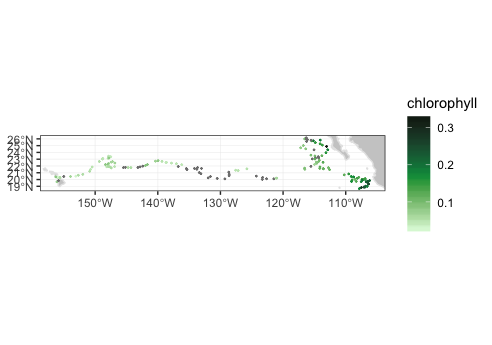### Animating the track

To make a cumulative animation of the track:

``````myPlot <- plotTrack(swchl1, xpos, ypos, tpos, plotColor = 'algae',
animate = TRUE, cumulative = TRUE)``````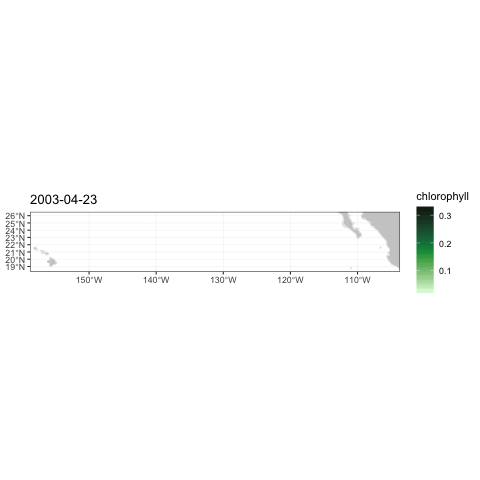marlin Track Animation

### Topography data

This example demonstrates how to pass a function to `plotTrack` to transform the data before plotting, how to change the name shown on the colorbar, and how to call `plotTrack()` if the dataset does not have a time coordinate.

``````require("ggplot2")
require("plotdap")
require("rerddap")
require("rerddapXtracto")
ylim <- c(15, 30)
xlim <- c(-160, -105)
topoInfo <- rerddap::info('etopo360')
topo <- rxtracto(topoInfo, parameter = 'altitude', xcoord = xpos, ycoord = ypos, xlen = .1, ylen = .1)
myFunc = function(x) -x
topoPlot <- plotTrack(topo, xpos, ypos, NA, plotColor = 'dense', name = 'Depth', myFunc = myFunc)
topoPlot``````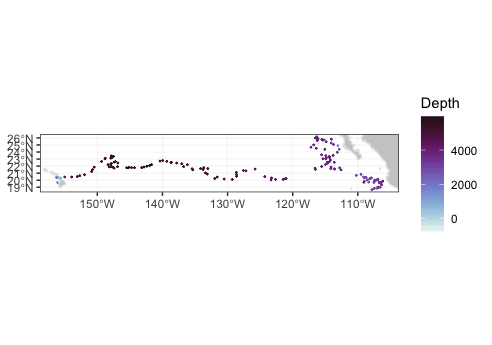### Track moving in (x, y, z, t) space.

The following is an artificial example showing a track moving in (x, y, z, t) space. Since the times of the model output change, the actual times are retrieved, and the last three times used in the example.

``````require("rerddap")
urlBase <- "https://erddap.marine.ie/erddap/"
parameter <- "Sea_water_temperature"
dataInfo <- rerddap::info("IMI_CONN_3D", url = urlBase)
#get the actual last 3 times,  and extract from data frame
sstTimes <- dataInfo1[]
sstLats <- c(53.505758092414446, 53.509303546859805, 53.51284900130517)
sstLons <- c(-10.25975390624996, -10.247847656249961, -10.23594140624996)
sstDepths <- c(2, 6, 10)
sstTrack <- rxtracto(dataInfo, parameter = parameter, xcoord = sstLons, ycoord = sstLats, tcoord = sstTimes, zcoord = sstDepths, xlen = .05, ylen = .05, zlen = 0., zName = 'altitude')
str(sstTrack)``````

This returns the following structure information:

``````List of 13
\$ mean Sea_water_temperature  : num [1:3] 11.7 11.7 11.6
\$ stdev Sea_water_temperature : num [1:3] 0.0407 0.0555 0.0859
\$ n                           : int [1:3] 493 491 484
\$ satellite date              : chr [1:3] "2020-10-31T22:00:00Z" "2020-10-31T23:00:00Z" "2020-11-01T00:00:00Z"
\$ requested lon min           : num [1:3] -10.3 -10.3 -10.3
\$ requested lon max           : num [1:3] -10.2 -10.2 -10.2
\$ requested lat min           : num [1:3] 53.5 53.5 53.5
\$ requested lat max           : num [1:3] 53.5 53.5 53.5
\$ requested z min             : num [1:3] 2 6 10
\$ requested z max             : num [1:3] 2 6 10
\$ requested date              : chr [1:3] "2020-10-31T22:00:00Z" "2020-10-31T23:00:00Z" "2020-11-01T00:00:00Z"
\$ median Sea_water_temperature: num [1:3] 11.7 11.7 11.6
\$ mad Sea_water_temperature   : num [1:3] 0.0208 0.0361 0.0986
- attr(*, "row.names")= chr [1:3] "1" "2" "3"
- attr(*, "class")= chr [1:2] "list" "rxtractoTrack"``````

### Crossing the dateline

The following is an artificial example of a track that crosses the date-line, using the MUR(Multi-scale Ultra-high Resolution) SST analysis:

``````dataInfo <- rerddap::info('jplMURSST41mday')
parameter <- 'sst'
xcoord <- c(179.7, 179.8, 179.9, 180., 180.1, 180.2, 180.3, 180.4)
ycoord <- c(40, 40, 40, 40, 40, 40, 40, 40)
tcoord <- c('2018-03-16', '2018-03-16', '2018-03-16','2018-03-16','2018-03-16','2018-03-16','2018-03-16','2018-03-16')
xlen <- .05
ylen <- .05
extract <- rxtracto(dataInfo, parameter = parameter, xcoord = xcoord,
ycoord = ycoord, tcoord = tcoord,
xlen = xlen, ylen = ylen)
str(extract)``````

The returned structure is:

``````List of 13
\$ mean sst         : num [1:8] 11.1 11.1 11.1 11.2 11.1 ...
\$ stdev sst        : num [1:8] 0.01192 0.00602 0.01025 0.00876 0.01446 ...
\$ n                : int [1:8] 30 30 35 25 30 30 30 35
\$ satellite date   : chr [1:8] "2018-03-16T00:00:00Z" "2018-03-16T00:00:00Z" "2018-03-16T00:00:00Z" "2018-03-16T00:00:00Z" ...
\$ requested lon min: num [1:8] 180 180 180 180 180 ...
\$ requested lon max: num [1:8] 180 180 180 180 180 ...
\$ requested lat min: num [1:8] 40 40 40 40 40 ...
\$ requested lat max: num [1:8] 40 40 40 40 40 ...
\$ requested z min  : logi [1:8] NA NA NA NA NA NA ...
\$ requested z max  : logi [1:8] NA NA NA NA NA NA ...
\$ requested date   : chr [1:8] "2018-03-16" "2018-03-16" "2018-03-16" "2018-03-16" ...
\$ median sst       : num [1:8] 11.1 11.1 11.1 11.2 11.1 ...
\$ mad sst          : num [1:8] 0.01416 0.0052 0.01149 0.00887 0.01744 ...
- attr(*, "row.names")= chr [1:8] "1" "2" "3" "4" ...
- attr(*, "class")= chr [1:2] "list" "rxtractoTrack"``````

## Using `rxtracto_3D`

The function `rxtracto_3D()` adds no new capabilities to `rerddap`, but returns the data as an array, rather than “melted” as does`rerddap::griddap()`. `rxtracto_3D()` also is used in the function `rxtractogon()`, so is provided for consistency. `rxtracto_3D()` also changes latitudes and longitudes to agree with those of the source ERDDAP dataset, and returns a structure where these are mapped back to the request.

### Obtaining VIIRS chlorophyll data

We examine VIIRS chlorophyll for the “latest” data as of when the vignette was generated:

``````require("rerddap")
require("rerddapXtracto")

xpos <- c(-125, -120)
ypos <- c(39, 36)
tpos <- c("last", "last")
tpos <- c("2017-04-15", "2017-04-15")
VIIRSInfo <- rerddap::info('erdVH3chlamday')
VIIRS <- rxtracto_3D(VIIRSInfo, parameter = 'chla', xcoord = xpos, ycoord = ypos, tcoord = tpos)``````

`rxtracto_3d()` returns a list of the form:

• VIIRS\$chla : num [xcoord, ycoord, tcoord]
• VIIRS\$datasetname: character string of the ERDDAP dataset ID
• VIIRS\$longitude : num [xcoord] longitude of extract
• VIIRS\$latitude : num [ycoord] latitudes of extract
• VIIRS\$altitude : num zcoord of extract
• VIIRS\$time : POSIXlt[tcoord] times of extract

The coordinate names of the structure are based on the names given in the `rxtracto_3d()` call, so may differ between datasets.

The extracted data can be mapped using using `plotBBox()`:

``````require("ggplot2")
require("plotdap")
myFunc <- function(x) log(x)
chlalogPlot <- plotBBox(VIIRS, plotColor = 'algae', myFunc = myFunc)
chlalogPlot``````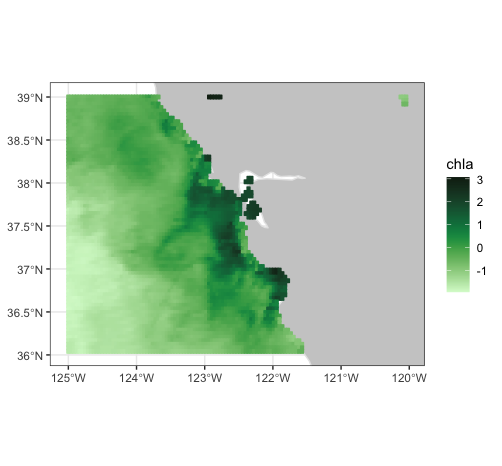### Crossing the date-line

The following is an `rxtracto_3D()` request that again uses the MUR dataset and crosses the date-line:

``````dataInfo <- rerddap::info('jplMURSST41mday')
parameter <- 'sst'
xcoord <- c(175, 185)
ycoord <- c(40, 50)
tcoord <- c('2019-03-16', '2019-03-16')
mur_dateline <- rxtracto_3D(dataInfo, parameter, xcoord = xcoord, ycoord = ycoord,
tcoord = tcoord)``````

## Plotting crossing the dateline

Plots that cross the date-line need to use the ‘world2’ continental outlines rather than the default. Due to some problems with that dataset, some regions must be removed in order to not get artificial lines.

``````xlim <- c(170, 190)
ylim <- c(40, 55)
remove <- c("UK:Great Britain", "France", "Spain", "Algeria", "Mali", "Burkina Faso", "Ghana", "Togo")
w <- map("world2Hires", xlim = xlim, ylim = ylim, fill = TRUE, plot = FALSE)
w <- map("mapdata::world2Hires", regions = w\$names[!(w\$names %in% remove)], plot = FALSE, fill = TRUE, ylim = ylim, xlim = xlim)``````

`plotBBox()` can plot across the date-line, but the x-axis labels can be incorrect. The data can be plotted using `ggplot2`. A function `mapFrame()` is defined to help melt the data into a dataframe suitable for `ggplot2`, and then plotted using the outline defined above.

``````mapFrame <- function(longitude, latitude, my_data) {
my_data_name <- names(my_data)
temp_data <- drop(my_data[])
dims <- dim(temp_data)
temp_data <- array(temp_data, dims * dims)
my_frame <- expand.grid(x = longitude, y = latitude)
my_frame[my_data_name] <- temp_data
return(my_frame)
}
mur_frame <- mapFrame(mur_dateline\$longitude, mur_dateline\$latitude, mur_dateline['sst'])
mycolor <- cmocean::cmocean('thermal')(256)
myplot <- ggplot(data = mur_frame, aes(x = x, y = y, fill = sst)) +
geom_polygon(data = w, aes(x = long, y = lat, group = group), fill = "grey80") +     geom_tile(interpolate = FALSE) +
scale_fill_gradientn(colours = mycolor, na.value = NA) +
theme_bw() + ylab("latitude") + xlab("longitude") +
coord_fixed(1.3, xlim = xlim, ylim = ylim)
myplot``````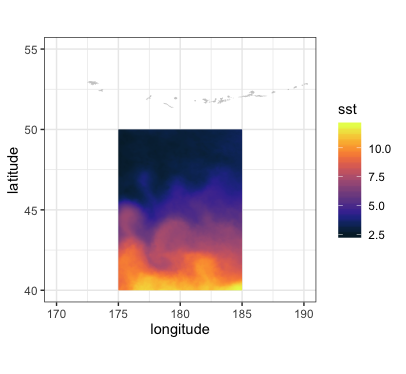## Using `rxtractogon`

The function `rxtractogon()` extracts a time-series of satellite data that are within a user supplied polygon. Two examples are given. The first extracts chlorophyll within the boundary points of the Monterey Bay National Marine Sanctuary, available in the `mbnms` dataset which is loaded with the `rerddapXtracto` package.

``````require("rerddapXtracto")
dataInfo <- rerddap::info('erdVH3chlamday')
parameter = 'chla'
tpos <- c("2014-09-01", "2014-10-01")
#tpos <-as.Date(tpos)
xpos <- mbnms\$Longitude
ypos <- mbnms\$Latitude
sanctchl <- rxtractogon(dataInfo, parameter = parameter, xcoord = xpos, ycoord = ypos,  tcoord = tpos)
str(sanctchl)``````

The returned structure is:

``````List of 6
\$ chla       : num [1:50, 1:57, 1:2] NA NA NA NA NA NA NA NA NA NA ...
\$ datasetname: chr "erdVH3chlamday"
\$ longitude  : num [1:50(1d)] -123 -123 -123 -123 -123 ...
\$ latitude   : num [1:57(1d)] 35.6 35.6 35.6 35.7 35.7 ...
\$ altitude   : logi NA
\$ time       : POSIXlt[1:2], format: "2014-09-15" "2014-10-15"
- attr(*, "class")= chr [1:2] "list" "rxtracto3D"``````

The extract (see `str(sanctchl)`) contains two time periods of chlorophyll masked for data only in the sanctuary boundaries. This example shows how to pull out only a single time period to be used in `plotBBox()`.

``````require("ggplot2")
require("plotdap")
myFunc <- function(x) log(x)
sanctchl1 <- sanctchl
sanctchl1\$chla <- sanctchl1\$chla[, , 2]
sanctchl1\$time <- sanctchl1\$time
sanctchlPlot <- plotBBox(sanctchl1, plotColor = 'algae', myFunc = myFunc)
sanctchlPlot``````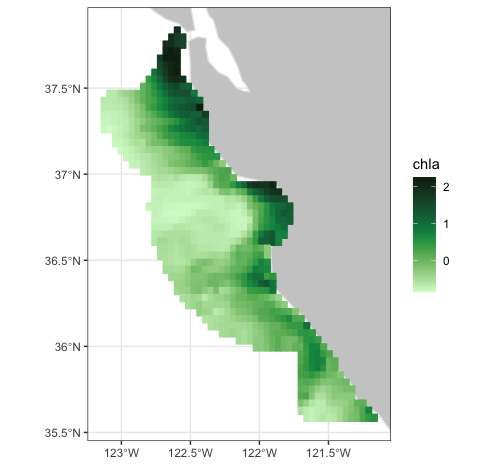The map of the extract can also be animated through time:

``````require("gganimate")
require("ggplot2")
require("plotdap")
myFunc <- function(x) log(x)
sanctchlPlot <- plotBBox(sanctchl, plotColor = 'algae', myFunc = myFunc, time = identity, animate = TRUE)``````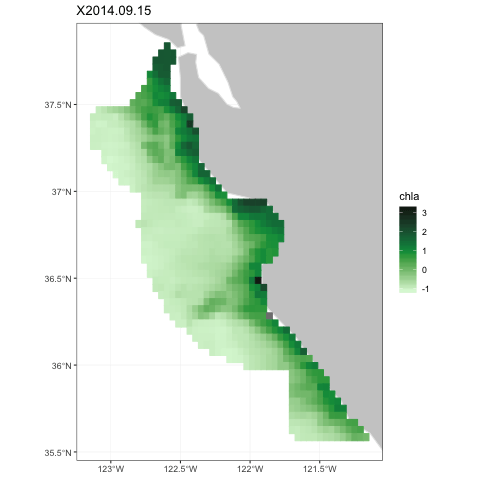Sanctuary Animation

The MBNMS is famous for containing the Monterey Canyon, which reaches depths of up to 3,600 m (11,800 ft) below surface level at its deepest. `rxtractogon()` can extract the bathymetry data for the MBNMS from the ETOPO dataset:

``````require("rerddap")
dataInfo <- rerddap::info('etopo180')
xpos <- mbnms\$Longitude
ypos <- mbnms\$Latitude
bathy <- rxtractogon(dataInfo, parameter = 'altitude', xcoord = xpos, ycoord = ypos)
str(bathy)``````

The returned structure is:

``````List of 6
\$ depth      : num [1:123, 1:141, 1] NA NA NA NA NA NA NA NA NA NA ...
\$ datasetname: chr "etopo180"
\$ longitude  : num [1:123(1d)] -123 -123 -123 -123 -123 ...
\$ latitude   : num [1:141(1d)] 35.5 35.6 35.6 35.6 35.6 ...
\$ altitude   : logi NA
\$ time       : logi NA
- attr(*, "class")= chr [1:2] "list" "rxtracto3D"``````

Mapping the data to show the canyon:

``````require("ggplot2")
require("mapdata")
myFunc = function(x) -x
bathyPlot <- suppressMessages((plotBBox(bathy, plotColor = 'dense', myFunc = myFunc, name = 'Depth')))
bathyPlot``````